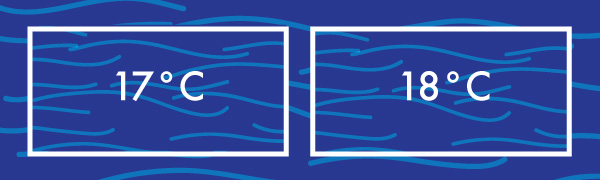# Global Warming and Expanding Ocean Volume

Algebra Level 3Scientist who have studied historical climate data believe that the oceans are increasing in temperature faster now that at any point in the last 10000 years. Over the last 60 years, the oceans have increased in temperature by $.18$ degrees C.

While this doesn't sound like much of a change, the density of water changes as its termperature increases. For example, water at 17 C has a density of $998.8$ kg/m$^3$, but water at 18 C has a density of $998.6$ kg/m$^3$. Assuming that in the temperature range we are considering the density function of water is roughly linear, how many more cubic km of ocean water on Earth are there now than there were 60 years ago? (give the nearest answer)

Assume that the current volume of the ocean is approximately $1.333 \times 10^9 \text{ km}^3.$

×

Problem Loading...

Note Loading...

Set Loading...